﻿ Free Math Worksheets and Printouts

# Free 2nd Grade Math Worksheets

## addition worksheets, subtraction worksheets, place value worksheets, money worksheets, time worksheets, measurement worksheets,......

###Single Digit Addition Worksheets

Common Core State Standards: 2.OA.2
Operations & Algebraic Thinking

Fluently add and subtract within 20 using mental strategies. 2 By end of Grade 2, know from memory all sums of two one-digit numbers.

###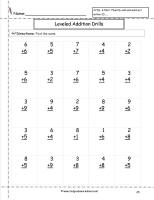Single Digit Addition Fluency Drills Worksheets

Common Core State Standards: 2.OA.2
Operations & Algebraic Thinking

Fluently add and subtract within 20 using mental strategies. 2 By end of Grade 2, know from memory all sums of two one-digit numbers.

###Three Single Digit Numbers Addition Worksheets

Common Core State Standards: 2.OA.2
Operations & Algebraic Thinking

Fluently add and subtract within 20 using mental strategies. 2 By end of Grade 2, know from memory all sums of two one-digit numbers.

###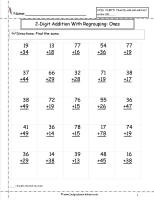Two Digit Addition Worksheets

Common Core State Standards: 2.NBT.5
Number & Operations in Base Ten
Use place value understanding and properties of operations to add and subtract.

Fluently add and subtract within 100 using strategies based on place value, properties of operations, and/or the relationship between addition and subtraction.

###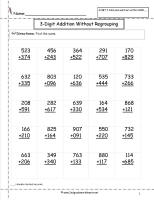Three Digit Addition Worksheets

Common Core State Standards: 2.NBT.7
Number & Operations in Base Ten
Use place value understanding and properties of operations to add and subtract.

Add and subtract within 1000, using concrete models or drawings and strategies based on place value, properties of operations, and/or the relationship between addition and subtraction; relate the strategy to a written method.......

###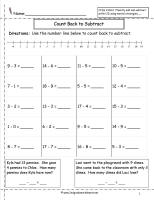Single Digit Subtraction Worksheets

Common Core State Standards: 2.OA.2
Operations & Algebraic Thinking

Fluently add and subtract within 20 using mental strategies. 2 By end of Grade 2, know from memory all sums of two one-digit numbers.

###Two Digit Subtraction Worksheets

Common Core State Standards: 2.NBT.5
Number & Operations in Base Ten
Use place value understanding and properties of operations to add and subtract.

Fluently add and subtract within 100 using strategies based on place value, properties of operations, and/or the relationship between addition and subtraction.

###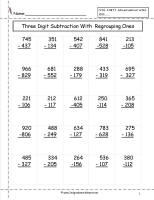Three Digit Subtraction Worksheets

Common Core State Standards: 2.NBT.7
Number & Operations in Base Ten
Use place value understanding and properties of operations to add and subtract.

Add and subtract within 1000, using concrete models or drawings and strategies based on place value, properties of operations, and/or the relationship between addition and subtraction; relate the strategy to a written method.......

###Place Value Worksheets

Common Core State Standards: 2.NBT.1
Number & Operations in Base Ten
Understand Place Value

Understand that the three digits of a three-digit number represent amounts of hundreds, tens, and ones; e.g., 706 equals 7 hundreds, 0 tens, and 6 ones.

###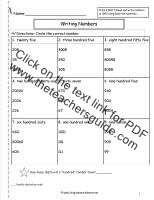Reading and Writing Numbers to 1000 Worksheets

Common Core State Standards: CCSS 2.NBT.3
Numbers & Operations in Base Ten
Understand place value.

Read and write numbers to 1000 using base ten numberals, number names, and expanded form.

###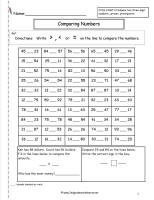Comparing Numbers Worksheets

Common Core State Standards: CCSS 2.NBT.4
Numbers & Operations in Base Ten
Understand place value.

Compare two three-digit numbers based on meanings of the hundreds, tens, and ones digits, using >, =, and < symbols to record the results of comparisons.

###Rounding Numbers Worksheets

Common Core State Standards: CCSS 3.NBT.1
Number & Operations in Base Ten
Use place value understanding and properties of operations to perform multi-digit arithmetic.

Use place value understanding to round whole numbers to the nearest 10 or 100.

###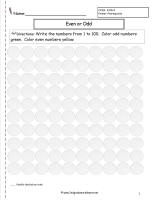Even and Odd Numbers Worksheets

Common Core State Standards: CCSS 2.OA.3 Primer, Prerequisite
Operations & Algebraic Thinking
Work with equal groups of objects to gain foundations for multiplication.

Determine whether a group of objects (up to 20) has an odd or even number of members, e.g., by pairing objects or counting them by 2s; write an equation to express an even number as a sum of two equal addends.

###Reading and Creating Bar Graphs Worksheets

Common Core State Standards: 2.MD.10
Measurement & Data
Represent and Interpret Data

Draw a picture graph and a bar graph (with single-unit scale) to represent a data set with up to four categories. Solve simple put-together, take-apart, and compare problems using information presented in a bar graph.

###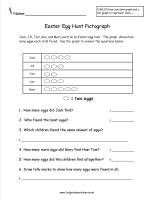Reading and Creating Pictograph Worksheets

Common Core State Standards: 2.MD.10
easurement & Data
Represent and Interpret Data

Draw a picture graph and a bar graph (with single-unit scale) to represent a data set with up to four categories. Solve simple put-together, take-apart, and compare problems using information presented in a bar graph.

###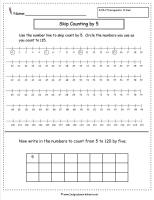Skip Counting Worksheets

Common Core State Standards: 2.NBT.2
Number & Operations in Base Ten
Understand place value.

Count within 1000, skip count by 5, 10, 100.

###Counting Money Worksheets

Common Core State Standards: 2.MD.8
Measurement & Data
Work with time and money.

Solve word problems involving dollar bills, quarters, dimes, nickels, and pennies, using \$ and ¢ symbols appropriately.

###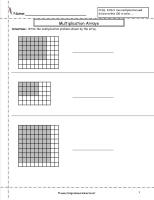Multiplication Arrays Worksheets

Common Core State Standards: Extension of CCSS 2.OA.4, CCSS 3.OA.3
Operations & Algebraic Thinking
Represent and solve problems involving multiplication and division.

Use multiplication and division within 100 to solve word problems in situations involving equal groups, arrays, and measurement quantities, e.g., by using drawings and equations with a symbol for the unknown number to represent the problem.

###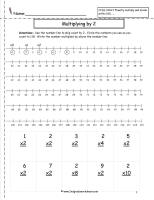Multiplication Facts Worksheets

Common Core State Standards: Extension of CCSS 2.OA.4, CCSS 3.OA.7
Operations & Algebraic Thinking
Multiply and divide within 100.

FFluently multiply and divide within 100, using strategies such as the relationship between multiplication and division (e.g., knowing that 8 × 5 = 40, one knows 40 ÷ 5 = 8) or properties of operations. By the end of Grade 3, know from memory all products of two one-digit numbers.

###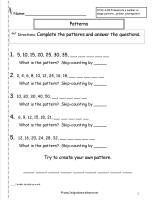Patterns Worksheets

Common Core State Standards: CCSS 4.OA.5
Operations and Algebraic Thinking
Generate and analyze patterns.

Generate a number or shape pattern that follows a given rule. Identify apparent features of the pattern that were not explicit in the rule itself.

###Telling Time Worksheets

Common Core State Standards: 2.MD.7
Measurement & Data
Work with time and money

Tell and write time from analog and digital clocks to the nearest five minutes, using a.m. and p.m.

###Fact Family Worksheets

Common Core State Standards: 2.OA.2
Operations & Algebraic Thinking